Study S1 Mathematics Maths - Number Patterns - Geniebook# Number Patterns

In this chapter, we will be discussing the below mentioned topics in detail:

• Common Difference (Direct)
• Common Difference (Indirect)
• General Term
• Find a formula for general term given a number pattern
• Find n given a particular iteration in the pattern
• Determine whether a given iteration is part of a number pattern

## Formula for the General Term, $$T_n$$

For any number sequence where each term differs from the successive term by a constant quantity,

$$T_n = a + d \;(n\; – 1)$$

Where, $$T_n$$ is the $$n^{th}$$ term in the sequence,

$$a$$ is the $$1^{st}$$ term in the sequence, and

$$d$$ is the common difference in quantity between successive terms.

Let’s understand this with the help of some examples:

Example 1:

Consider the following number sequence:

$$1^{st}\;term$$                   $$2^{nd}\;term$$                $$3^{rd}\;term$$                $$4^{th}\;term$$                $$5^{th}\;term$$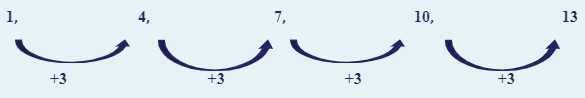For this sequence, it starts with $$1$$, then $$+3$$ to each term to get the next term.

\begin{align*} T_n &= 1 + 3 (n\; – 1)\\ &=1 + 3 n\; – 3\\ &=3n-2 \end{align*}

## Number Patterns

A number pattern is a sequence of figures linked by a specific rule.

Example 1:

Consider the following number pattern: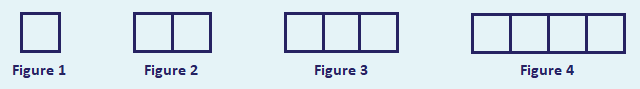How would the next two figures look like?

Solution: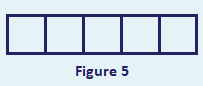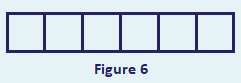#### Number Of Lines

$$1$$ $$1$$ $$4$$

$$2$$

$$2$$

$$4 + 3 = 7$$

$$3$$

$$3$$

$$4 + 3 + 3 = 10$$

$$4$$

$$4$$

$$4 + 3 + 3 + 3 = 13$$

$$5$$

$$5$$

$$4 + 3 \;(4) = 16$$

$$6$$

$$6$$

$$4 + 3 \;(5) = 19$$

$$n$$

$$n$$

\begin{align*} 4 + 3(n – 1) &= 4 + 3n – 3\\ &= 3n + 1 \end{align*}

Hence, the number of lines in the $$n^{th}$$ figure, $$L_n = 3n + 1$$

Example 2:

The diagram shows some patterns made from floor tiles.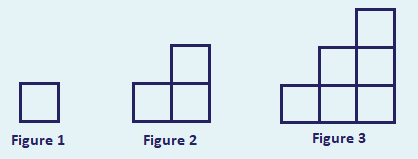Find an expression, in terms of $$n$$, for the total number of tiles, $$T_n$$, in Figure $$n$$.

Solution:

#### Number Of Tiles

$$1$$

\begin{align*} 1 && && && && =1 && && = \frac{1\times2}{2} \end{align*}

$$2$$

\begin{align*} 1+2 && && && =3 && = \frac{2\times3}{2} \end{align*}

$$3$$

\begin{align*} 1+2+3 && && =6 && = \frac{3\times4}{2} \end{align*}

$$4$$

\begin{align*} 1+2+3+4 &&=10 && = \frac{4\times5}{2} \end{align*}

In Figure $$n$$

$$T_n = \frac{n \;(n+1)}2$$

Question 1:

The International Space Station (ISS) consists of oval shaped Space Pods and rectangular Solar Panels. The first 3 iterations of the ISS are as shown.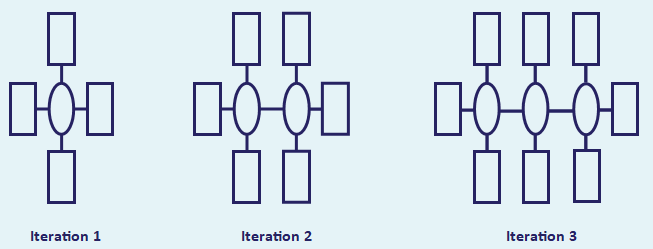Find a formula, in terms of $$n$$, for

1. the total number of Space Pods, $$A$$, in iteration $$n$$, and
2. the total number of Space Panels, $$n$$, in iteration $$n$$.

Solution:

1. In Iteration 1, there is $$1$$ Space Pod.

In Iteration 2, there are $$2$$ Space Pods and so on.

Hence, in Iteration n, the number of Space Pods would be $$A = n$$

1. In Iteration 1, there are $$4$$ Space Panels; in Iteration 2, there are $$6$$ Space Panels; and in Iteration 3, there are $$8$$ Space Panels and so on.

Hence, in Iteration n, the number of Space Panels would be

\begin{align*} B &= 4 + 2 \;(n \;– 1)\\ &= 4 + 2n \;– 2\\ &= 2n + 2 \end{align*}

Continue Learning
Basic Geometry Linear Equations
Number Patterns Percentage
Prime Numbers Ratio, Rate And Speed
Functions & Linear Graphs 1 Integers, Rational Numbers And Real Numbers
Basic Algebra And Algebraic Manipulation 1 Approximation And EstimationPrimaryPrimary 1Primary 2Primary 3Primary 4Primary 5Primary 6SecondarySecondary 1EnglishMaths
Basic Geometry
Linear Equations
Number Patterns
Percentage
Prime Numbers
Ratio, Rate And Speed
Functions & Linear Graphs 1
Integers, Rational Numbers And Real Numbers
Basic Algebra And Algebraic Manipulation 1
Approximation And EstimationScienceSecondary 2Secondary 3Secondary 4